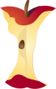Home > Grade Levels > Grade 2 >

## Addition and Subtraction (Within 20) Word Problems#### Aligned To Common Core Standard:

Grade 2 Operations- 2.OA.2

### Printable Worksheets And Lessons

• Step-by-Step Lesson- Two digit adding and subtracting. Somewhere in between we include visuals of single digits too.

• Guided Lesson - Operations puzzles that look for sums and differences. Followed by missing addends and subtrahends problems.

• Guided Lesson Explanation - For the puzzles, I didn't go into too much detail because as another standard at this level points out, students should master these skills to 1000. So single digits should be a breeze.

• Practice Worksheet - Mixed problems with input / output boxes of sums and differences under 20.

• Matching Problems to Answers Worksheet - Find the missing addends and subtrahends by matching them to where they are missing from.

###(Click Here to Upgrade)

#### Homework Sheets

We show you how to do one and you do the rest.

#### Practice Worksheets

Students are asked to look at the questions in a slightly different way with this one.

#### Math Skill Quizzes

A whole mess of quizzes for you to have fun with.

 Quiz 1 Quiz 2 Quiz 3 Quiz 4 Quiz 5 Quiz 6 Quiz 7 Quiz 8